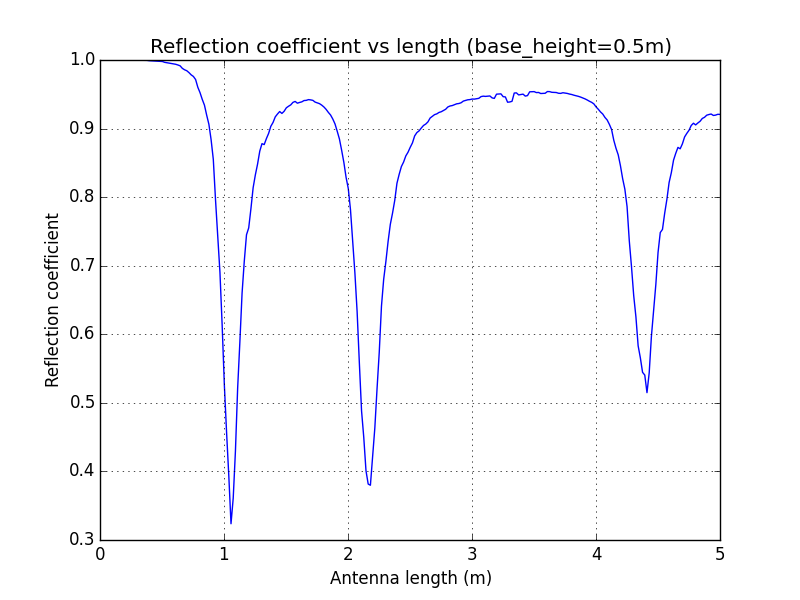## Introduction to Modelling antennas with python-necpp

Nec2++ is a program for simulating antennas. It is a rewrite in C++ of an old FORTRAN code called NEC2 and has some nice features. In particular it provides a library and an API so that simulations can be done in other languages. A python module (python-necpp) has just been developed that allows you to simulate antennas using nec2++.

### Installation

Installation is easy with the python pip installer.  Install python-necpp with

```    pip install necpp
```

This will download and compile the python-necpp package.

### A Simple Antenna

NEC2 was based on punch cards and an antenna model was described as a series of Cards. These are well documented. Nec2++ replaces these cards with function calls each function call the equivalent of an nec2 card -- here's an example.

We'll model a vertical monopole (like a whip antenna on an old radio).

```from necpp import *
import math

def handle_nec(result):
if (result != 0):
print nec_error_message()

def monopole_impedance(freq, base, length):
wavelength = 3e8/(1e6*freq)
n_seg = int(math.ceil(50*length/wavelength))
nec = nec_create()
handle_nec(nec_wire(nec, 1, n_seg, 0, 0, base, 0, 0, base+length, 0.002, 1.0, 1.0))
handle_nec(nec_geometry_complete(nec, 1, 0))
handle_nec(nec_fr_card(nec, 0, 1, freq, 0))
handle_nec(nec_ex_card(nec, 0, 0, n_seg/3, 0, 1.0, 0, 0, 0, 0, 0))
handle_nec(nec_xq_card(nec, 0)) # Execute simulation
# Results
z = complex(nec_impedance_real(nec,0), nec_impedance_imag(nec,0))
# Cleanup
nec_delete(nec)
return z

z = monopole_impedance(freq=134.5, base=0.1, length=4.0)
print "f=134.5 z = (%6.1f,%+6.1fI) Ohms" % (z.real, z.imag)
```

When you run this code, it will print out the impedance of the antenna:

```\$ python quarter_wave.py
f=134.5 z = ( 141.0,-416.2I) Ohms
```

### Impedance Mismatch

Radio recevers and transmitters are designed to operate with antennas of a specific impedance (z0). If the antenna has a different impedance (z), this impedance mismatch causes loss of signal. The reflection coefficient measures how much signal is reflected at the junction between the antenna and the radio.  The reflection coefficient (Gamma) is given by
```def reflection_coefficient(z, z0):
return np.abs((z - z0) / (z + z0))
```
The transmission coefficient is (1.0 - Gamma) and represents how much of the original signal makes it through this junction.

### Searching for an optimum antenna

Imagine the antenna is fixed 0.5 meters above the ground and we are connecting the signal to the antenna one-third of the way along its length. How long should the wire be for optimum performance?

If we minimize the reflection coefficient, this is a relatively easy optimization. We can use matplotlib to plot the reflection coefficient as a function of length, with the base_height of the antenna fixed.
```import numpy as np
import pylab as plt

lengths = np.linspace(0.2, 5.0, 270)
reflections = []
z0 = 50

for l in lengths:
z = monopole_impedance(freq=134.5, base=0.5, length=l)
reflections.append(reflection_coefficient(z, z0))

plt.plot(lengths, reflections)
plt.xlabel("Antenna length (m)")
plt.ylabel("Reflection coefficient")
plt.title("Reflection coefficient vs length (base_height=0.5m)")
plt.grid(True)
plt.show()
```The reflection coefficient has several minima when the base height is 0.5m. The first occurs when the antenna length is just over 1m.
This shows that for short lengths, less than 10 percent of the signal makes it through. There is a local minimum (of approximately 0.3) that occurs around 1.1m for which around 70 percent of the signal makes it through.

### Next Steps

In another post I'll show how to use python-necpp and matplotlib to automatically design a vehicle antenna.

### Links

#### 5 comments:

1.When i try to run this code on Lubuntu 14.04, it drops an error "NameError: global name 'nec_create' is not defined", i tried others codes from GitHub and got the same result, any suggest?, I installed the package necpp, and python-necpp from synaptic.

2.I have been a regular visitor of this site and I love reading blogs posted here.They are truly very well written,precise and to the point. Thanks.

cell phone signal booster antenna & gps antenna manufacturer

3.Doing the pip install, I get:

> error: can't copy '../necpp_src/example/test.py': doesn't exist or not a regular file

4.The link to http://www.nec2.org/part_3/cards/toc.html is not very useful, as all the links on that page are broken.

5.This code is written in python2 style. Is python3 supported?